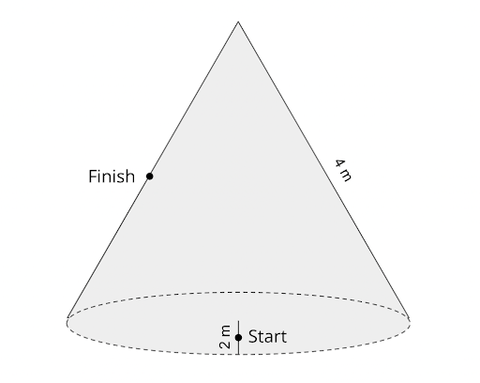# Riddler Classic Puzzle 3-04-2022

Two ants named Geo and Desik are racing along the surface of a cone. The circular base of the cone has a radius of 2 meters and a slant height of 4 meters. Geo and Desik both start the race on the base, a distance of 1 meter away from its center.

The race’s finish is halfway up the cone, 90 degrees around the cone’s central axis from the start, as shown in the following diagram:Geo and Desik both want your help in strategizing for the race. What is the length of the shortest path from the start to the finish?

FiveThirtyEight

SOLUTION

If we unfurl the cone to get a semicircle of radius 4, we have the following diagram:For every angle θ the circular base 'rotates' from its initial position, the point of tangency moves an angle of θ/2 around the semicircle.  Since the Finish is located at a quarter of the semicircle (45°)  the resulting angle is 45° - θ/2.

Law of Cosines gives the total distance from Start to Finish:

√(12 + 2- 4 cos θ) + √[22 + 4- 16 cos (45° - θ/2)]

We can try to minimize this by calculus, but a less unwieldy method is to notice that if Start to Finish is the shortest path, we expect it to be a straight line

So the triangle with sides 1, 2, angle θ  should be similar to the triangle with sides  2, 4, angle 45° - θ/2.

Thus,  45° - θ/2  =  θ

Or θ = 30°

Plugging θ = 30° above gives:

3 √(5 - 2√3)  ≈  3.718